Ex 14.2

Chapter 14 Class 7 Symmetry
Serial order wise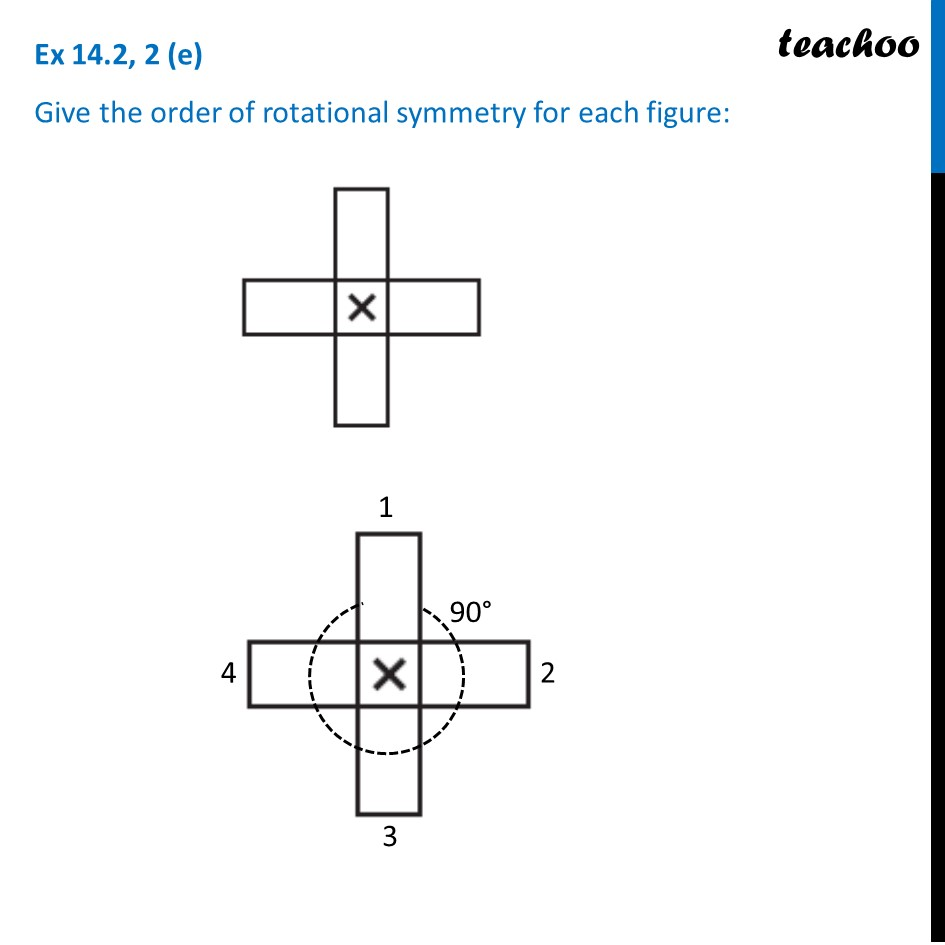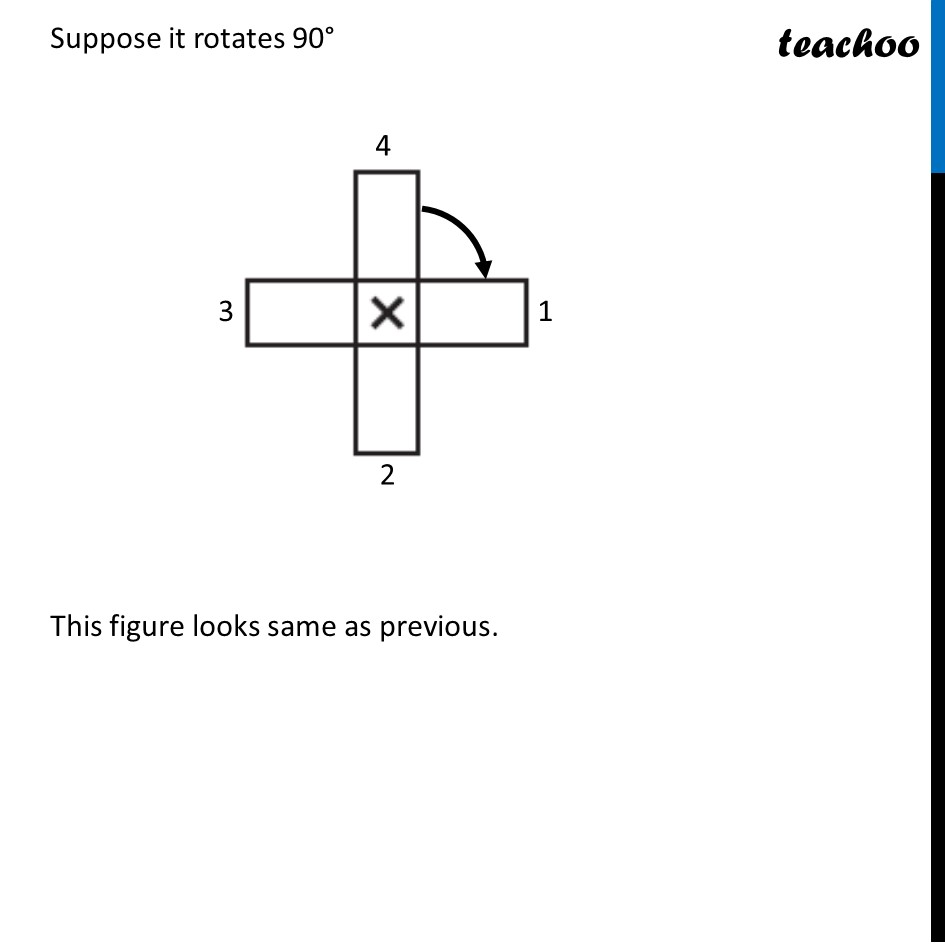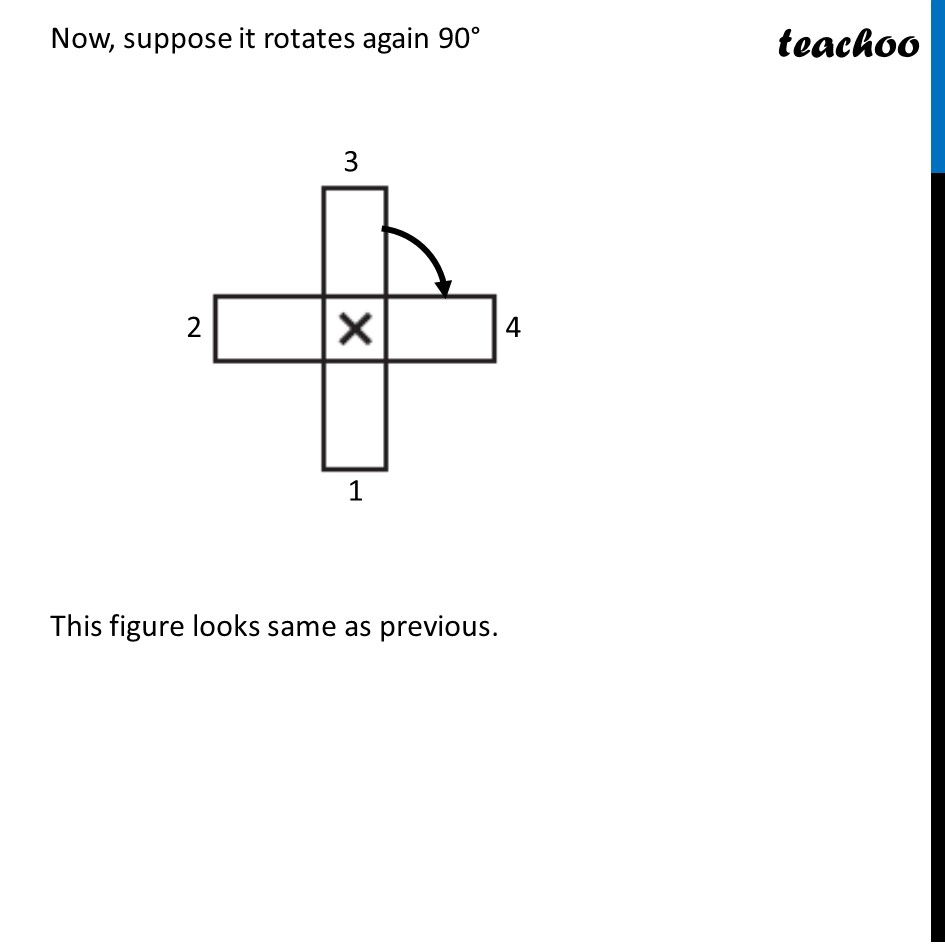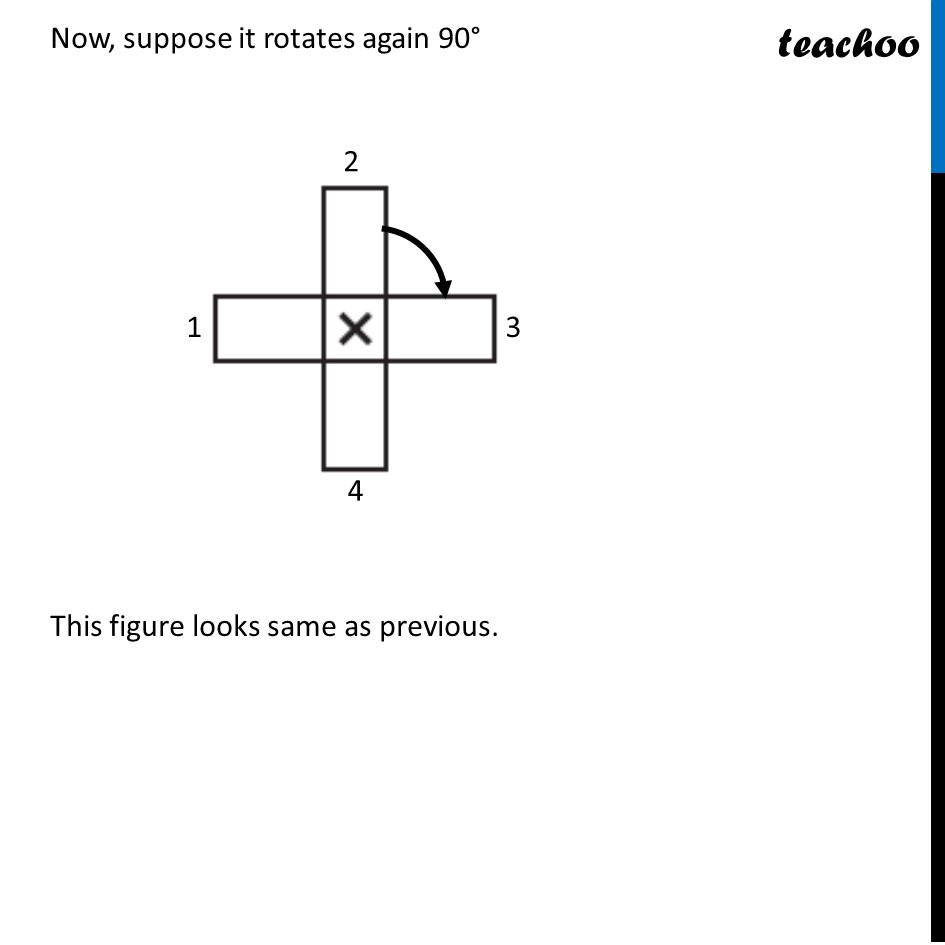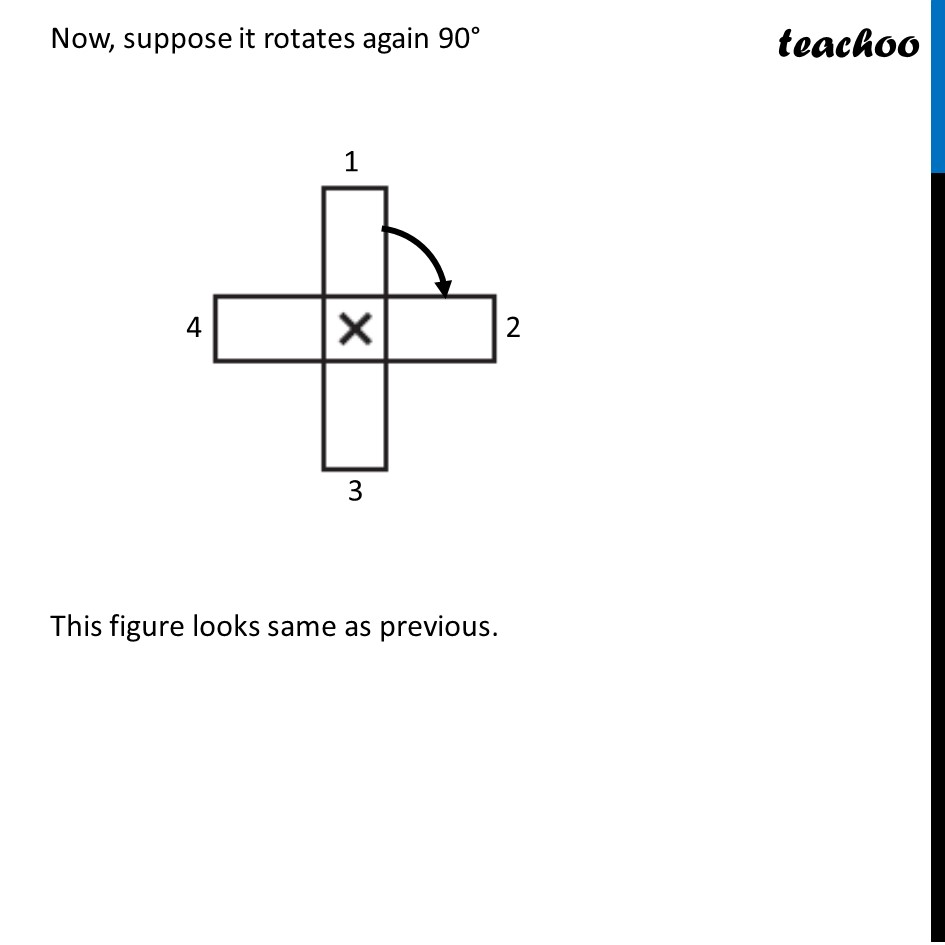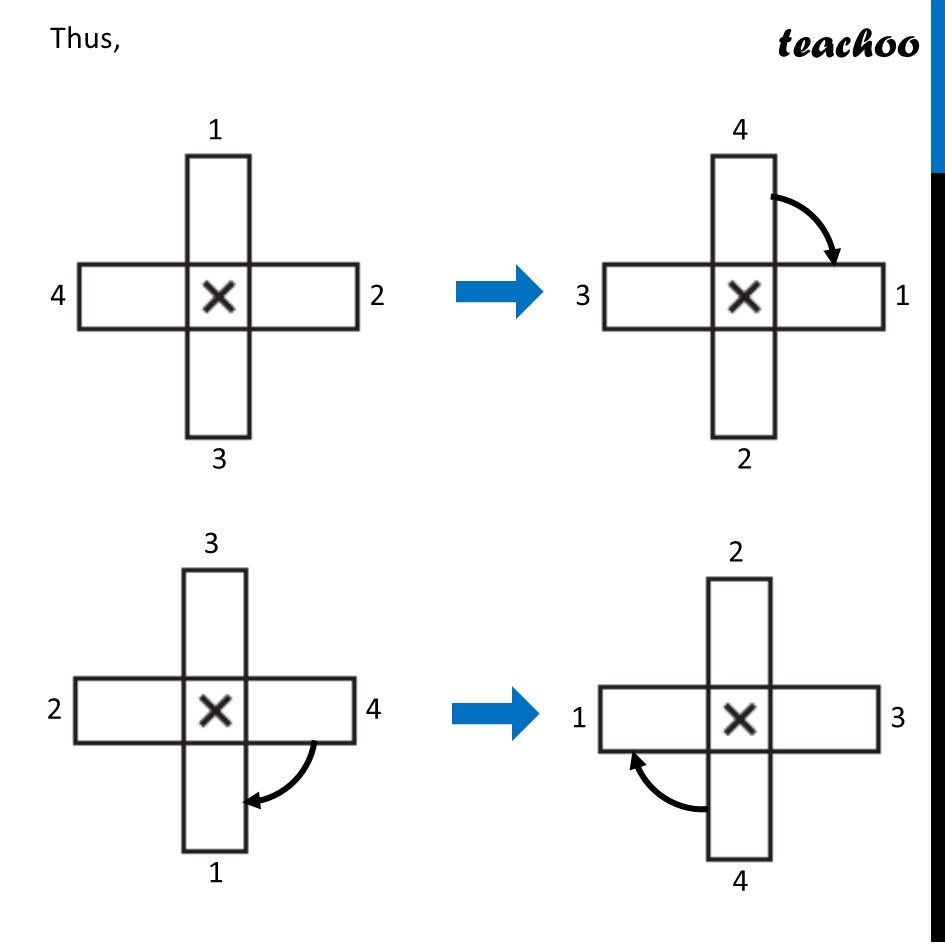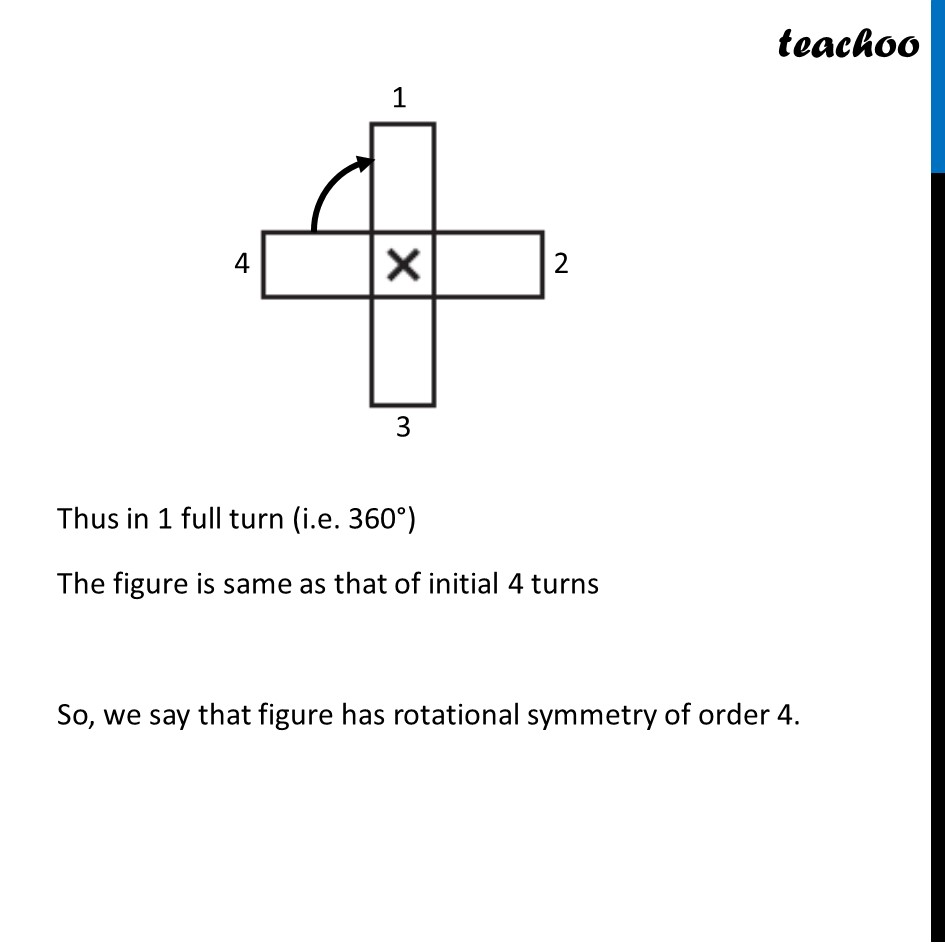### Transcript

Ex 14.2, 2 (e) Give the order of rotational symmetry for each figure: Suppose it rotates 90° This figure looks same as previous. Now, suppose it rotates again 90° This figure looks same as previous. Now, suppose it rotates again 90° This figure looks same as previous. Now, suppose it rotates again 90° This figure looks same as previous. Thus, Thus in 1 full turn (i.e. 360°) The figure is same as that of initial 4 turns So, we say that figure has rotational symmetry of order 4.